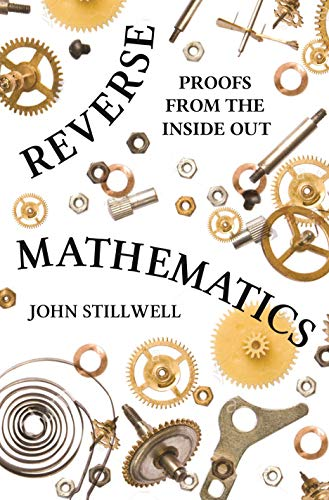Free Shipping in Australia
Proud to be B-Corp

# Reverse Mathematics John Stillwell\$38.99
Condition - Very Good
Only 1 left

## Reverse Mathematics Summary

### Reverse Mathematics: Proofs from the Inside Out by John Stillwell

This book presents reverse mathematics to a general mathematical audience for the first time. Reverse mathematics is a new field that answers some old questions. In the two thousand years that mathematicians have been deriving theorems from axioms, it has often been asked: which axioms are needed to prove a given theorem? Only in the last two hundred years have some of these questions been answered, and only in the last forty years has a systematic approach been developed. In Reverse Mathematics, John Stillwell gives a representative view of this field, emphasizing basic analysis--finding the right axioms to prove fundamental theorems--and giving a novel approach to logic. Stillwell introduces reverse mathematics historically, describing the two developments that made reverse mathematics possible, both involving the idea of arithmetization. The first was the nineteenth-century project of arithmetizing analysis, which aimed to define all concepts of analysis in terms of natural numbers and sets of natural numbers. The second was the twentieth-century arithmetization of logic and computation. Thus arithmetic in some sense underlies analysis, logic, and computation. Reverse mathematics exploits this insight by viewing analysis as arithmetic extended by axioms about the existence of infinite sets. Remarkably, only a small number of axioms are needed for reverse mathematics, and, for each basic theorem of analysis, Stillwell finds the right axiom to prove it. By using a minimum of mathematical logic in a well-motivated way, Reverse Mathematics will engage advanced undergraduates and all mathematicians interested in the foundations of mathematics.

## Reverse Mathematics Reviews

If you are not familiar with this relatively new research about the foundations and and minimal assumptions needed to develop the massive mathematical structure, this provides a good informal guideline.---Adhemar Bultheel, European Mathematical Society
John Stillwell's book gives a clear and engaging introduction to an intriguing area of mathematics: reverse mathematics.---Martyn Prigmore, Mathematics Today
The book is rich in examples and historical perspectives, is clearly argued and immaculately presented.---Graham Hoare, Mathematical Gazette

John Stillwell is professor of mathematics at the University of San Francisco and an affiliate of the School of Mathematical Sciences at Monash University, Australia. His many books include Mathematics and Its History and Elements of Mathematics: From Euclid to Godel (Princeton).

GOR009012814
9780691177175
0691177171
Reverse Mathematics: Proofs from the Inside Out by John Stillwell
Used - Very Good
Hardback
Princeton University Press
20180101
200
N/A
Book picture is for illustrative purposes only, actual binding, cover or edition may vary.
This is a used book - there is no escaping the fact it has been read by someone else and it will show signs of wear and previous use. Overall we expect it to be in very good condition, but if you are not entirely satisfied please get in touch with us